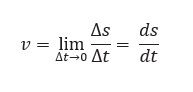The time rate of change of position of the object in any direction is called the speed of the object.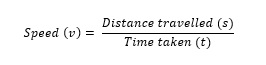Its unit is m/s. It is a scalar quantity.
Its dimensional formula is [M0 T-1].

## Uniform Speed:

If an object covers equal distances in equal intervals of time, then its speed is called uniform speed.

## Non-Uniform Speed or Variable Speed:

If an object covers unequal distances in equal intervals of time, then its speed is called non-uniform or variable speed.

## Average Speed:

The ratio of the total distance traveled by the object to the total time taken is called the average speed of the object.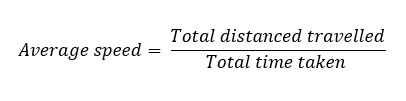If a particle travels distances s1 , s2 , s3 …sn with speeds v1 , v2 , v3 …vn then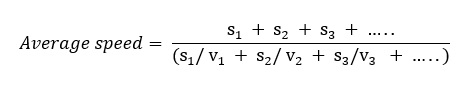If particle travels equal distances ( s1 = s2 = s) with velocities v1 and v2 , then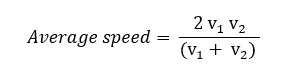If a particle travels with speeds v1, v2, v3 …vn, during time intervals t1, t2, t3 …tn then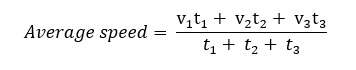If particle travels with speeds v1 and v2  for equal time intervals, i.e., t1 =t2, =t3 then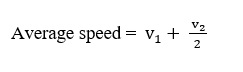When a body travels equal distance with speeds v1 and v2  the average speed (v) is the
harmonic mean of two speeds.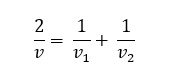## Instantaneous Speed:

When an object is traveling at a variable speed, then its speed at a given instant of time is called its instantaneous speed.